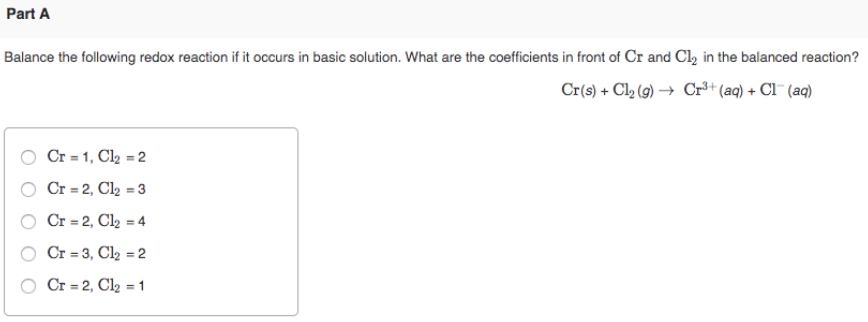# Balance the following redox reaction if it occurs in basic solution. What are the coefficients in front of Cr and Cl2 in the balanced reaction? Cr(s) + Cl2(g) → Cr^3+(aq) + Cl^-(aq) a) Cr = 1, Cl2 = 2 b) Cr = 2, Cl^2 = 3 c) Cr = 2, Cl2 = 4 d) Cr = 3, Cl2 =2 e) Cr = 2, Cl2 = 1